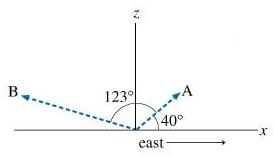# A radar station, located at the origin of xz plane, as shown in the figure , detects an airplane coming straight at the station from the east.Dolly Robinson 2021-01-13 Answered

A radar station, located at the origin of xz plane, as shown in the figure , detects an airplane coming straight at the station from the east. At first observation (point A), the position of the airplane relative to the origin is $\stackrel{\to }{{R}_{A}}$. The position vector $\stackrel{\to }{{R}_{A}}$ has a magnitude of ${360}^{m}$ and is located at exactly ${40}^{\circ }$ above the horizon. The airplane is tracked for another ${123}^{\circ }$} in the vertical east-west plane for ${5.0}^{s}$, until it has passed directly over the station and reached point B. The position of point B relative to the origin is $\stackrel{\to }{{R}_{B}}$ (the magnitude of $\stackrel{\to }{{R}_{B}}$ is ${880}^{m}$). The contact points are shown in the diagram, where the x axis represents the ground and the positive z direction is upward.Define the displacement of the airplane while the radar was tracking it: ${\stackrel{\to }{R}}_{BA}={\stackrel{\to }{R}}_{B}-{\stackrel{\to }{R}}_{A}$. What are the components of ${\stackrel{\to }{R}}_{BA}$
Express ${\stackrel{\to }{R}}_{BA}$ in meters as an ordered pair, separating the x and z components with a comma, to two significant figures.

You can still ask an expert for help

• Questions are typically answered in as fast as 30 minutes

Solve your problem for the price of one coffee

• Math expert for every subject
• Pay only if we can solve itsovienesY

The position vector $\stackrel{\to }{{R}_{A}}$ is given by
${\stackrel{\to }{R}}_{A}=\left(r\mathrm{cos}\theta \right)\stackrel{^}{i}+\left(r\mathrm{sin}\theta \right)\stackrel{^}{k}$
Substitute (360m) for r and 40 deegres for $\theta$ to find $\stackrel{\to }{{R}_{A}}$
${\stackrel{\to }{R}}_{A}=\left(\left(360m\right)\mathrm{cos}{40}^{\circ }\right)\stackrel{^}{i}+\left(\left(360m\right)\mathrm{sin}{40}^{\circ }\right)\stackrel{^}{k}$
$=\left(275.8m\right)\stackrel{^}{i}+\left(231.4m\right)\stackrel{^}{k}$
The position vector $\stackrel{\to }{{R}_{B}}$ is given by,
${\stackrel{\to }{R}}_{B}=\left(r\mathrm{cos}\theta \right)\stackrel{^}{i}+\left(r\mathrm{sin}\theta \right)\stackrel{^}{k}$
The angle for the position vector $over\to {\left\{R\right\}}_{B}$ along vertical and horizontal plane is,
$\theta ={123}^{\circ }+{40}^{\circ }={163}^{\circ }$
Substitute (880m) for r and 163 deegres for $\theta$ to find $\stackrel{\to }{{R}_{B}}$
${\stackrel{\to }{R}}_{B}=\left(\left(880m\right)\mathrm{cos}{163}^{\circ }\right)\stackrel{^}{i}+\left(\left(880m\right)\mathrm{sin}{163}^{\circ }\right)\stackrel{^}{k}$
$=\left(-841.5m\right)\stackrel{^}{i}+\left(257.3m\right)\stackrel{^}{k}$
The resultant displacement vectors components of the air plane are, ${\stackrel{\to }{R}}_{BA}={\stackrel{\to }{R}}_{B}-{\stackrel{\to }{R}}_{A}$
Substitute $\left(-841.5m\right)\stackrel{^}{i}+\left(257.3m\right)\stackrel{^}{k}$ for $\stackrel{\to }{{R}_{B}}$ and $\left(275.8m\right)\stackrel{^}{i}+\left(231.4m\right)\stackrel{^}{k}$ for $\stackrel{\to }{{R}_{A}}$ to find $\stackrel{\to }{{R}_{BA}}$.
${\stackrel{\to }{R}}_{BA}=\left(-841.5m\right)\stackrel{^}{i}+\left(257.3m\right)\stackrel{^}{k}-\left(275.8m\right)\stackrel{^}{i}+\left(231.4m\right)\stackrel{^}{k}$
$=-\left(1117.3m\right)\stackrel{^}{i}+\left(25.9m\right)\stackrel{^}{k}$ Round off the components of resultant displacement vectors in to two significant figures, ${\stackrel{\to }{R}}_{BA}=-\left(1100m\right)\stackrel{^}{i}+\left(26m\right)\stackrel{^}{k}$
Therefore, the displacement of the airplane as an ordered pair separated by the x and z components is (-1100m,26m).

###### Not exactly what you’re looking for?Jeffrey Jordon

Vector A:

$\mathrm{cos}40=\frac{x}{360}$; x = 276

$\mathrm{sin}{40}^{\circ }=\frac{y}{360}$; y = 231

${R}_{{A}_{x}},{R}_{{A}_{z}}=\left(275.775,231.40\right)$

Vector B:

$\mathrm{\angle }\left(123+40=163;180-163={17}^{\circ }\right)$

${R}_{{B}_{x}},{R}_{{B}_{z}}=\left(-841.55,257.29\right)$

${R}_{BA}=\stackrel{\to }{B}-\stackrel{\to }{A}=\left(-841.55,257.29\right)-\left(275.775,231.40\right)=\left(-116.84,25.89\right)$